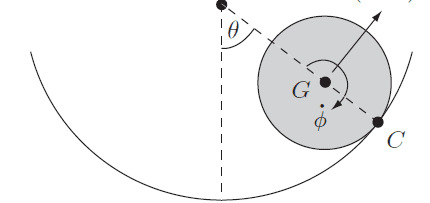# Motion of Rolling Cylinder in Fixed Cylinder: Confusing Constraint Condition

• LCSphysicist
In summary, the conversation discusses the problem of finding the equations of motion for a cylinder inside a fixed cylinder, as it rolls and rotates without slipping. The constraint condition for non-slipping is equivalent to saying that the circumference traced by a point on the small cylinder's perimeter must be equal to the distance traced by the body itself. This leads to the equation (b-a)θ = aϕ, where θ represents the rotation of the small cylinder and ϕ represents its rotation about its own axis. The conversation also includes a visual representation of the motion.f

#### LCSphysicist

Homework Statement
.
Relevant Equations
,The problem is a classical one, basically to find the equations of motion of cylinder of radius a inside a fixed cylinder of radius b, the cylinder that rolls rotate about its own axis in such way that it does not skid/slip.

Now, the thing that is making myself confused is the constraint condition: I know the answer is ##(b-a) \dot \theta = a \dot \phi##, but i can't understand it! Aat least to me, the condition of non slipping is equivalent to say the circunference traced by a point initially at the contact with the rough surface is equal to the distance traced by the body itself. So, the cylinder moved ##b \theta## while it rotates ##a \phi##. So the condition, to me, could be written as ##(b) \dot \theta = a \dot \phi##. I don't understand why it is being used (b-a) instead! That is, this is the distance traveled by the CG, but shouldn't we seek for the distance traveled by the point of contact?

So, the cylinder moved bθ while it rotates aϕ.
No.
Start by imagining the small cylinder rotating through dϕ but its centre remaining fixed (so it is slipping). adϕ of its perimeter skids across a fixed point on the outer cylinder. Call the point on its perimeter in contact with the outer cylinder P.
Now move the small cylinder, without further rotation, to where it would have been had it rolled instead. If it was rotating clockwise then this involves moving it anticlockwise.
Note that the point of contact is no longer P. Instead, it is adθ anticlockwise around from P. So length of perimeter of the small cylinder that would have come into contact during rolling is adϕ+adθ.

(I assume you know that the easy way is to consider the small cylinder rotating about its point of contact, so its centre moves through an arc length (b-a)dθ.)

•andresB and LCSphysicist
I don't understand why it is being used (b-a) instead! That is, this is the distance traveled by the CG, but shouldn't we seek for the distance traveled by the point of contact?
Thought this might be worth adding as the animation below is fun to watch (well, it is for me).

Starting with ##\theta = \phi = 0##, after some rolling we have ##(b-a)\theta = a\phi## (not ##b\theta = a\phi##).

To see what is happening, suppose ##a = 1## and ##b = 4##. This give ##\phi = 3\theta## (not ##\phi = 4\theta##). For example when point G does one full ‘orbit’ (##\theta = 2\pi##) then the small cylinder performs 3 (not 4) full rotations (##\phi = 6\pi## not ##8\pi##).

Watch it actually happen in the link below! Count the number of rotations of the small circle by noting the orientation of its radius. The small circle rotates only 3 times. (Warning: might make your eyes go funny!):
https://en.wikipedia.org/wiki/File:Astroid2.gif

•LCSphysicist and andresB